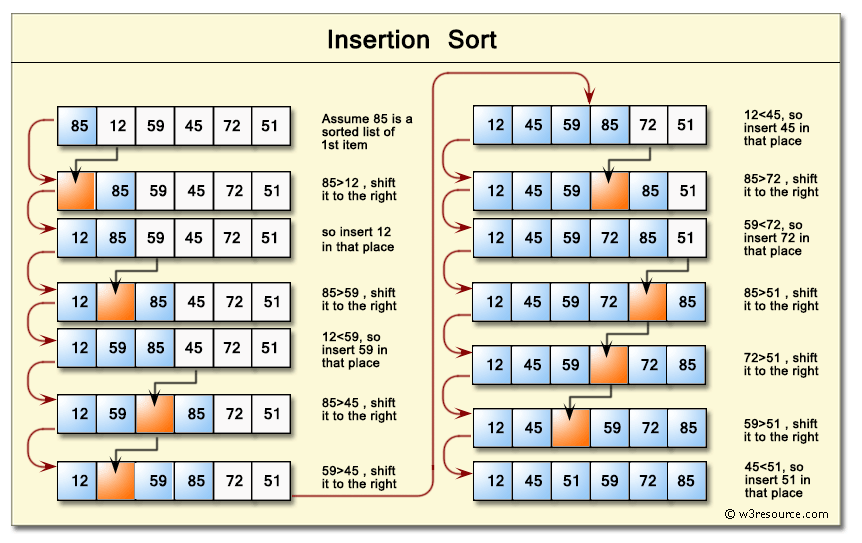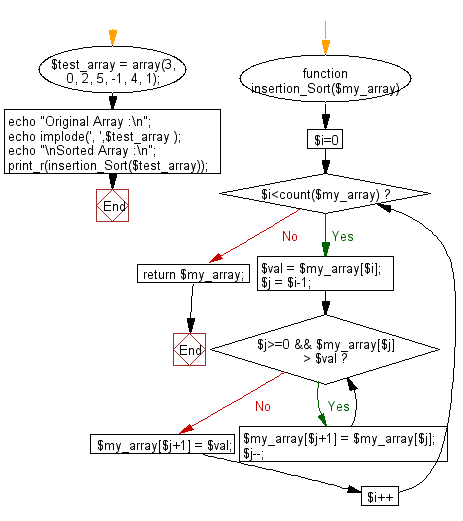﻿ PHP: Sort a list of elements using Insertion sort - w3resource# PHP Searching and Sorting Algorithm: Insertion sort

## PHP Searching and Sorting Algorithm: Exercise-3 with Solution

Write a PHP program to sort a list of elements using Insertion sort.

Insertion sort is a simple sorting algorithm that builds the final sorted array (or list) one item at a time. It is much less efficient on large lists than more advanced algorithms such as quicksort, heapsort, or merge sort.

Pictorial Presentation : Insertion SortA graphical example of insertion sort :Animation Credits : Swfung8

Sample Solution :

PHP Code :

``````<?php

function insertion_Sort(\$my_array)
{
for(\$i=0;\$i<count(\$my_array);\$i++){
\$val = \$my_array[\$i];
\$j = \$i-1;
while(\$j>=0 && \$my_array[\$j] > \$val){
\$my_array[\$j+1] = \$my_array[\$j];
\$j--;
}
\$my_array[\$j+1] = \$val;
}
return \$my_array;
}
\$test_array = array(3, 0, 2, 5, -1, 4, 1);
echo "Original Array :\n";
echo implode(', ',\$test_array );
echo "\nSorted Array :\n";
print_r(insertion_Sort(\$test_array));
?>
```
```

Sample Output:

```Original Array :
3, 0, 2, 5, -1, 4, 1
Sorted Array :
Array
(
 => -1
 => 0
 => 1
 => 2
 => 3
 => 4
 => 5
)
```

Flowchart :PHP Code Editor:

Have another way to solve this solution? Contribute your code (and comments) through Disqus.

What is the difficulty level of this exercise?

﻿

## PHP: Tips of the Day

Convert HTML + CSS to PDF

Important: Please note that this answer was written in 2009 and it might not be the most cost-effective solution today in 2019. Online alternatives are better today at this than they were back then.

Here are some online services that you can use:

• PDFShift
• Restpack
• PDF Layer
• DocRaptor
• HTMLPDFAPI
• HTML to PDF Rocket

Have a look at PrinceXML.

It's definitely the best HTML/CSS to PDF converter out there, although it's not free (But hey, your programming might not be free either, so if it saves you 10 hours of work, you're home free (since youalso need to take into account that the alternative solutions will require you to setup a dedicated server with the right software).

Ref : https://bit.ly/384yytr